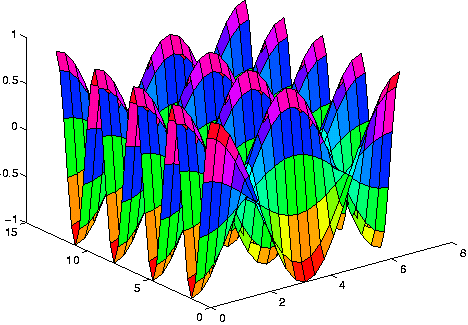# Definition: MATLAB

(MATrix LABoratory) A programming language for technical computing from The MathWorks, Natick, MA (www.mathworks.com). Used for a wide variety of scientific and engineering calculations, especially for automatic control and signal processing, MATLAB runs on Windows, Mac and a variety of Unix-based systems. Developed by Cleve Moler in the late 1970s and based on the original LINPACK and EISPACK FORTRAN libraries, it was initially used for factoring matrices and solving linear equations. Moler commercialized the product with two colleagues in 1984. MATLAB is also noted for its extensive graphics capabilities. The following MATLAB commands generate the 3D graph below:

```     x=(0:2*pi/20:2*pi)';
y=(0:4*pi/40:4*pi)';
[X,Y] = meshgrid(x,y);
z= cos(X).*cos(2*Y);
surf (x,y,z);
```The Resulting Graph
This graph was computed from the lines of code above and is an example that might be used in multivariate calculus. (Image courtesy of Dr. Mark S. Gockenbach, Michigan Technological University, www.math.mtu.edu/~msgocken/intro/intro.html)